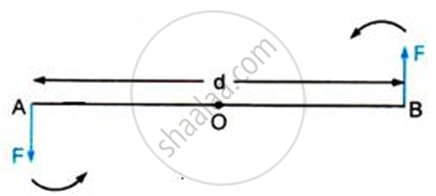Share

# Draw a Neat Labelled Diagram to Show the Direction of Two Forces Acting on a Body to Produce Rotation in It. Also, Mark the Point About Which Rotation Takes Place by the Letter O - ICSE Class 10 - Physics

ConceptElementary Introduction of Translational and Rotational Motions

#### Question

Draw a neat labelled diagram to show the direction of two forces acting on a body to produce rotation in it. Also, mark the point about which rotation takes place by the letter O.

#### SolutionAt A and B, two equal and opposite forces each of magnitude F are applied. The two forces rotate the bar in the anticlockwise direction.

Is there an error in this question or solution?
Solution Draw a Neat Labelled Diagram to Show the Direction of Two Forces Acting on a Body to Produce Rotation in It. Also, Mark the Point About Which Rotation Takes Place by the Letter O Concept: Elementary Introduction of Translational and Rotational Motions.
S